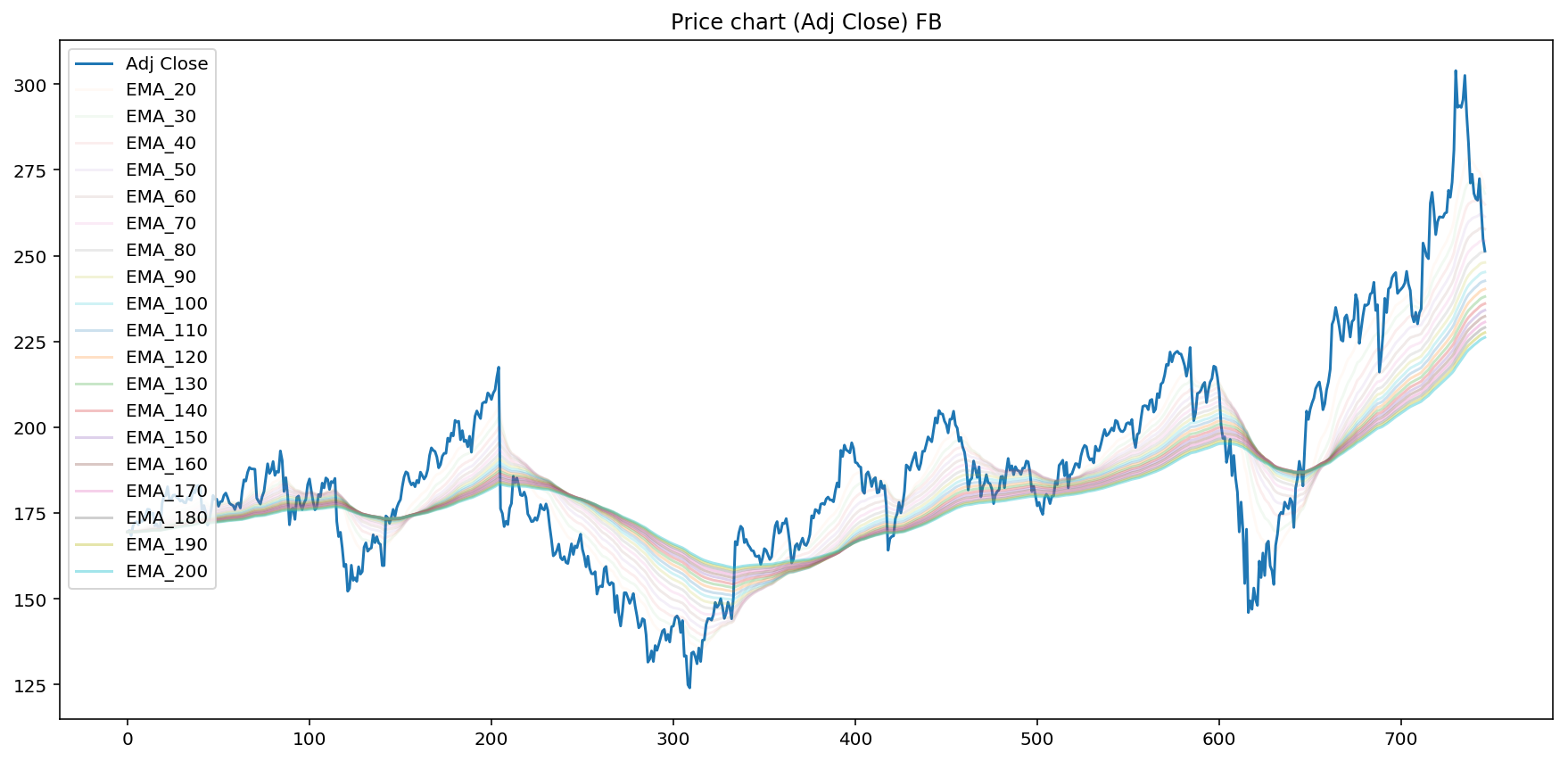## Turbulent waves - multiple moving averages¶

In technical analysys for stock price evaluation there are few significant moving averages that tend to work as rather strong support and resistance levels.

Some of the popular ones are 21, 50, 100, 150, 200 datapoint (day, hour) moving averages.

But there is alternative approach. We can create series of multiple moving averages and plot them alongside the price chart.

When price is approaching these multiple layers from above, they act as support. And conversely when price is below them, they act as resistance. The more layers the price goes through, the more significant support/resistance becomes.

In this script we are using exponentially weighted moving average (EMA).

In :
from IPython.core.display import display, HTML
display(HTML("<style>.container { width:100% !important; }</style>"))
%matplotlib inline

from IPython.display import set_matplotlib_formats
set_matplotlib_formats('retina')

In :
import sys
import os

# ___library_import_statements___
import pandas as pd

# for pandas_datareader, otherwise it might have issues, sometimes there is some version mismatch
pd.core.common.is_list_like = pd.api.types.is_list_like

# make pandas to print dataframes nicely
pd.set_option('expand_frame_repr', False)

import numpy as np
import matplotlib.pyplot as plt
import datetime
import time

import yfinance as yahoo_finance

In :
#                           ___variables___
# ------------------------------------------------------------------------------

#ticker = 'AAPL'
#ticker = 'TSLA'
ticker = 'FB'
#ticker = 'AMZN'
#ticker = 'SPY'
#ticker = 'QQQ'

start_time = datetime.datetime(2017, 10, 1)
#end_time = datetime.datetime(2019, 1, 20)
end_time = datetime.datetime.now().date().isoformat()     # today

In :
#                       __function_definitions__
# ------------------------------------------------------------------------------

def get_data(ticker):
# yahoo gives only daily historical data
attempts = 0
connected = False
while not connected:
try:
ticker_df = web.get_data_yahoo(ticker, start=start_time, end=end_time)
connected = True
print('connected to yahoo')
except Exception as e:
print("type error: " + str(e))
time.sleep( 5 )
attempts += 1
if attempts >= 10:
connected = True
pass

# use numerical integer index instead of date
ticker_df = ticker_df.reset_index()

return ticker_df

In :
def computeEMA(data, span):
# simple moving average

return ema

In :
def construct_df(ticker):
#get data from yahoo API
df = get_data(ticker)
# compute both types of moving averages
for i in range(20, 210, 10):
#print(i)

return df


In :
def plot_data_EMA(df):
plt.figure(figsize=(15,7))
plt.title('Price chart (Adj Close) ' + ticker)

for i in range(20, 210, 10):
#
alpha = i / 500.
plt.plot(df['EMA_{}'.format(i)], alpha=alpha)

plt.legend(loc='upper left')
plt.show()

In :
df = construct_df(ticker)

connected to yahoo

In :
plot_data_EMA(df)